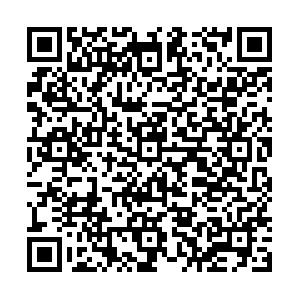EI、Scopus 收录

## 留言板引用本文: 周碧柳, 靳艳飞. 高斯色噪声和谐波激励共同作用下耦合SD振子的混沌研究. 力学学报, 待出版Zhou Biliu, Jin Yanfei. Chaos research of coupled sd oscillator under gaussian colored noise and harmonic excitation. Chinese Journal of Theoretical and Applied Mechanics, in press doi: 10.6052/0459-1879-22-123
 Citation: Zhou Biliu, Jin Yanfei. Chaos research of coupled sd oscillator under gaussian colored noise and harmonic excitation. Chinese Journal of Theoretical and Applied Mechanics, in press## 高斯色噪声和谐波激励共同作用下耦合SD振子的混沌研究

##### doi: 10.6052/0459-1879-22-123

###### 作者简介:靳艳飞, 教授, 主要研究方向: 非线性随机动力学. E-mail:jinyf@bit.edu.cn
• 中图分类号: O313.1

## CHAOS RESEARCH OF COUPLED SD OSCILLATOR UNDER GAUSSIAN COLORED NOISE AND HARMONIC EXCITATION

• 摘要: 耦合SD振子作为一种典型的负刚度振子, 在工程设计中有广泛应用. 同时高斯色噪声广泛存在于外界环境中, 并可能诱发系统产生复杂的非线性动力学行为, 因此其随机动力学是非线性动力学研究的热点和难点问题. 本文研究了高斯色噪声和谐波激励共同作用下双稳态耦合SD振子的混沌动力学, 由于耦合SD振子的刚度项为超越函数形式, 无法直接给出系统同宿轨道的解析表达式, 给混沌阈值的分析造成了很大的困难. 为此, 本文首先采用分段线性近似拟合该振子的刚度项, 发展了高斯色噪声和谐波激励共同作用下的非光滑系统的随机Melnikov方法. 其次, 基于随机Melnikov过程, 利用均方准则和相流函数理论分别得到了弱噪声和强噪声情况下该振子混沌阈值的解析表达式, 讨论了噪声强度对混沌动力学的影响. 研究结果表明, 随着噪声强度的增大混沌区域增大, 即增大噪声强度更容易诱发耦合SD振子产生混沌. 当阻尼一定时, 弱噪声情况下混沌阈值随噪声强度的增加而减小; 但是强噪声情况下噪声强度对混沌阈值的影响正好相反. 最后, 数值结果表明, 利用文中的方法研究高斯色噪声和谐波激励共同作用下耦合SD振子的混沌是有效的.本文的结果为随机非光滑系统的混沌动力学研究提供了一定的理论指导.

• 图  1  耦合SD振子的结构示意图

Figure  1.  The structural diagram of a coupled SD oscillator

图  2  函数$F(x)$$\tilde F(x)$的变化曲线.

Figure  2.  The plot of functions $F(x)$and $\tilde F(x)$图  3  势函数$V(x)$Figure  3.  The plot of the potential function $V(x)$图  4  哈密顿方程的相图.(a)$H(x,y) = E$;(b) $\tilde H(x,y) = E$Figure  4.  Phase portrait is plotted via Hamiltonian equation. (a)$H(x,y) = E$;(b)$\tilde H(x,y) = E$图  5  系统(10)同宿轨道附近的稳定流形与不稳定流形

Figure  5.  Stable and unstable manifolds near Homoclinic orbit of system (10)

图  6  弱噪声情形下系统(7)的混沌阈值

Figure  6.  Chaos threshold for system (7) with weak noise

图  7  弱噪声情形下在$\omega = 0.8$$c = 0.02$处系统(7)的混沌阈值

Figure  7.  Chaos threshold for system (7) with weak noise at $\omega = 0.8$and $c = 0.02$8  弱噪声情形原系统(1)的混沌

8.  Chaos for the original system (1) with weak noise

8  弱噪声情形原系统(1)的混沌(续)

8.  Chaos for the original system (1) with weak noise (continued)

9  弱噪声情形分段系统(7)的混沌

9.  Chaos for the piecewise linear system (7) with weak noise

图  10  固定$D$$\omega$时系统在弱噪声情形的混沌阈值

Figure  10.  Chaos threshold for system with weak noise when $D$and $\omega$are fixed

图  11  强噪声情形下系统(7)的混沌阈值

Figure  11.  Chaos threshold for system (7) with strong noise

图  12  强噪声情形下在$\omega = 5.2$$c = 0.05$处系统(7)的混沌阈值

Figure  12.  Chaos threshold for system (7) with strong noise at $\omega = 5.2$and $c =$0.05

图  13  强噪声情形原系统(1)的混沌

Figure  13.  Chaos for the original system (1) with strong noise

14  强噪声情形分段系统(7)的混沌

14.  Chaos for the piecewise linear system (7) with strong noise

14  强噪声情形分段系统(7)的混沌(续)

14.  Chaos for the piecewise linear system (7) with strong noise (continued)

图  15  固定$D$$\omega$时系统在强噪声情形的混沌阈值

Figure  15.  Chaos threshold for system with strong noise when $D$and $\omega$are fixed

•点击查看大图
##### 计量
• 文章访问数:  17
• HTML全文浏览量:  6
• PDF下载量:  2
• 被引次数: 0
##### 出版历程
• 收稿日期:  2022-03-24
• 录用日期:  2022-05-11
• 网络出版日期:  2022-05-10

### 目录/下载:  全尺寸图片 幻灯片
• 分享
• 用微信扫码二维码

分享至好友和朋友圈